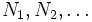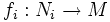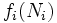# Difference between revisions of "Manifold is not union of images of manifolds of smaller dimension"

Let$M$ be a smooth manifold and$N_1, N_2, \ldots$ be a countable sequence of smooth manifolds, each having dimension strictly less than that of$M$. Suppose$f_i:N_i \to M$ are smooth maps. Then,$M$ is not the union of$f_i(N_i)$.